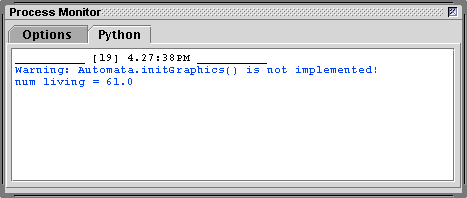Basic Cellular Automata Python Classes

Introduction

This tutorial is part of a series that deal with the issues of programming cellular automata (CA) for use with Maya. This tutorial presents two python classes that implement the core functionality of a system that represents a 2D cellular automata. The classes will require subclassing in order to create graphics of the type described in Wikipedia's "Cellular Automaton".

Instances of the Cell class (listing 1) encapsulate the following data,
- the state of the cell ie. alive or dead,
- the previous state of the cell,
- the number of times the cell has been alive.
The initial state of a cell is decided by chance. Instances of the Cell class do not maintain any information about their own graphical representation or the state of their neighbors. It is left to subclasses of the Cell class to implement the code that creates a Maya shape that will represent the cell and its state.

An instance of the Automata class is responsible for,
- creating multiple instances of the Cell class, or instances of a subclass of Cell,
- maintaining a two dimensional list of cell instances ie. a list of lists of cells,
- applying Conways "rules of life" to determine the state of each cell,
Like the Cell class, the Automata class must be subclassed in order to create visually interesting results.

Listing 1 (AbstractAutomata.py)

 import random class Cell: def __init__(self, chance): self.prevState = 0 self.state = 0 self.lived = 0 if random.random() <= chance: self.prevState = 1 self.state = 1 self.lived += 1 def getState(self): return self.state ## Subclasses will override this method in order to ## update the appearance of the shape that represents ## a cell. def setState(self, state): self.state = state if state == 1: self.lived += 1 def getPrevState(self): return self.prevState def setPrevState(self, state): self.prevState = state def copyState(self): self.prevState = self.state def getNumLived(self): return self.lived #______________________________________________________________ class Automata: def __init__(self, numcols, numrows, chanceOfLife): self.rows = numrows self.cols = numcols self.numcells = numrows * numcols self.cells = [] # Make a (column) list. for i in range(numcols): column = [] # Make a list of (row) cells for each column for j in range(numrows): cell = self.getACell(chanceOfLife) column.append(cell) self.cells.append(column) self.initGraphics() ## Subclasses will override this method so that ## instances of their subclass of Cell will be used by ## the automata. def getACell(self, chanceOfLife): return Cell(chanceOfLife) ## Subclasses will override this method in order to ## position the shapes that represent the cells. def initGraphics(self): print("Warning: Automata.initGraphics() is not implemented!") def getAllCells(self): return self.cells def countLiving(self): numLiving = 0.0 for i in range(self.cols): for j in range(self.rows): numLiving += self.cells[i][j].getState() return numLiving def nextGeneration(self): # Move to the "next" generation for i in range(self.cols): for j in range(self.rows): self.cells[i][j].copyState() for i in range(self.cols): for j in range(self.rows): numLive = 0 iprev = i - 1 inext = i + 1 jprev = j - 1 jnext = j + 1 # When a cell is at the outer edge we "wrap" if i == 0: iprev = self.cols - 1 if j == 0: jprev = self.rows - 1 if i == self.cols - 1: inext = 0 if j == self.rows - 1: jnext = 0 # Query the state of the neighborhood. # Cells those above___ numLive += self.cells[iprev][jprev].getPrevState() numLive += self.cells[ i ][jprev].getPrevState() numLive += self.cells[inext][jprev].getPrevState() # Cells either side___ numLive += self.cells[iprev][ j ].getPrevState() numLive += self.cells[inext][ j ].getPrevState() # Cells below___ numLive += self.cells[iprev][jnext].getPrevState() numLive += self.cells[ i ][jnext].getPrevState() numLive += self.cells[inext][jnext].getPrevState() prevState = self.cells[i][j].getPrevState() currCell = self.cells[i][j] Automata.applyRulesOfLife(self, currCell, numLive) ## Subclasses can override this method in order to apply ## their own custom rules of life. def applyRulesOfLife(self, cell, liveNeighbors): if cell.prevState == 1: if liveNeighbors == 2 or liveNeighbors == 3: cell.setState(1) else: cell.setState(0) if cell.prevState == 0: if liveNeighbors == 3: cell.setState(1) else: cell.setState(0)

Testing the Classes

Save the contents of listing 1 in a file named AbstractAutomata.py. The file should be saved in a directory that will be sourced by Maya. For example, it could be saved in,

maya/scripts

Alternatively, if the reader has followed the suggestions outlined in Maya: Setup for Python the file should be saved in,

maya/projects/RMS_python

Listing 2 (TestAbstractClasses.py) provides the code for a script that will test the Cell and Automata classes. It should also be saved in the same directory as AbstractAutomata.py. Open the script in Cutter and run it by using the keyboard shortcut control + e, alt + e or apple + e. Cutter's Process Monitor should show text similar to that displayed in figure 1.

Listing 2 (TestAbstractClasses.py)

 import AbstractAutomata as abstract automata = abstract.Automata(10, 10, 0.5) for n in range(1): num = automata.countLiving() print("num living = %s" % num) automata.nextGeneration()Figure 1

The next tutorial, "Algorithmic Form: CA PolyPlanes" demonstrates how Cell and Automata can be subclassed and used by Maya to display an animation of the "interaction" of the cells of a cellular automata.